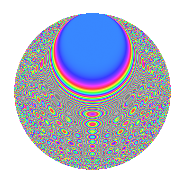# Properties

 Label 2100.2.dhLevel 2100 Weight 2 Character orbit dh Rep. character $$\chi_{2100}(109,\cdot)$$ Character field $$\Q(\zeta_{30})$$ Dimension 320 Sturm bound 960

# Related objects

## Defining parameters

 Level: $$N$$ $$=$$ $$2100 = 2^{2} \cdot 3 \cdot 5^{2} \cdot 7$$ Weight: $$k$$ $$=$$ $$2$$ Character orbit: $$[\chi]$$ $$=$$ 2100.dh (of order $$30$$ and degree $$8$$) Character conductor: $$\operatorname{cond}(\chi)$$ $$=$$ $$175$$ Character field: $$\Q(\zeta_{30})$$ Sturm bound: $$960$$

## Dimensions

The following table gives the dimensions of various subspaces of $$M_{2}(2100, [\chi])$$.

Total New Old
Modular forms 3936 320 3616
Cusp forms 3744 320 3424
Eisenstein series 192 0 192

## Trace form

 $$320q - 2q^{5} - 40q^{9} + O(q^{10})$$ $$320q - 2q^{5} - 40q^{9} - 6q^{11} - 4q^{15} - 20q^{17} - 8q^{19} - 12q^{25} + 24q^{29} + 6q^{31} - 20q^{35} + 36q^{41} + 2q^{45} + 100q^{53} + 40q^{55} - 24q^{59} + 28q^{61} + 10q^{63} + 26q^{65} + 32q^{69} + 32q^{71} + 40q^{73} + 8q^{75} + 12q^{79} + 40q^{81} + 40q^{83} + 148q^{85} + 58q^{91} + 38q^{95} + 20q^{97} + 8q^{99} + O(q^{100})$$

## Decomposition of $$S_{2}^{\mathrm{new}}(2100, [\chi])$$ into newform subspaces

The newforms in this space have not yet been added to the LMFDB.

## Decomposition of $$S_{2}^{\mathrm{old}}(2100, [\chi])$$ into lower level spaces

$$S_{2}^{\mathrm{old}}(2100, [\chi]) \cong$$ $$S_{2}^{\mathrm{new}}(175, [\chi])$$$$^{\oplus 6}$$$$\oplus$$$$S_{2}^{\mathrm{new}}(350, [\chi])$$$$^{\oplus 4}$$$$\oplus$$$$S_{2}^{\mathrm{new}}(525, [\chi])$$$$^{\oplus 3}$$$$\oplus$$$$S_{2}^{\mathrm{new}}(700, [\chi])$$$$^{\oplus 2}$$$$\oplus$$$$S_{2}^{\mathrm{new}}(1050, [\chi])$$$$^{\oplus 2}$$

## Hecke characteristic polynomials

There are no characteristic polynomials of Hecke operators in the database